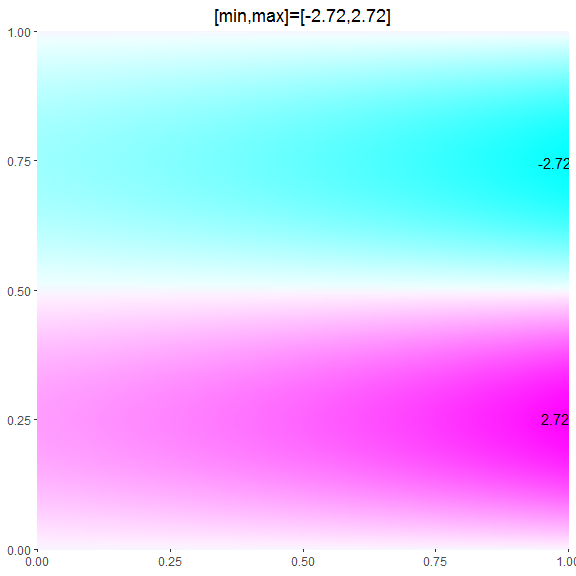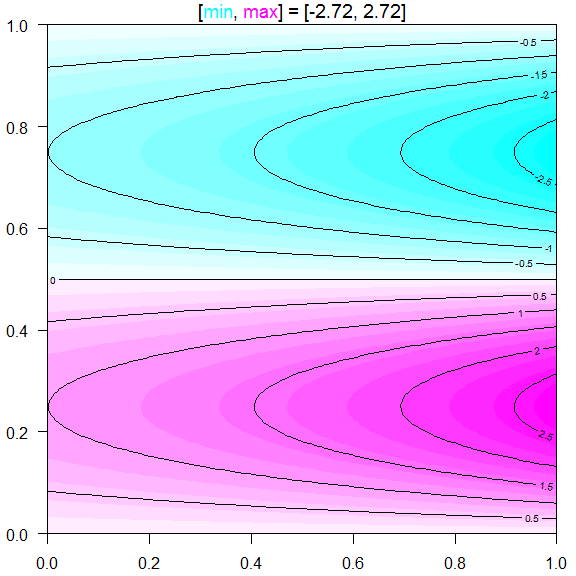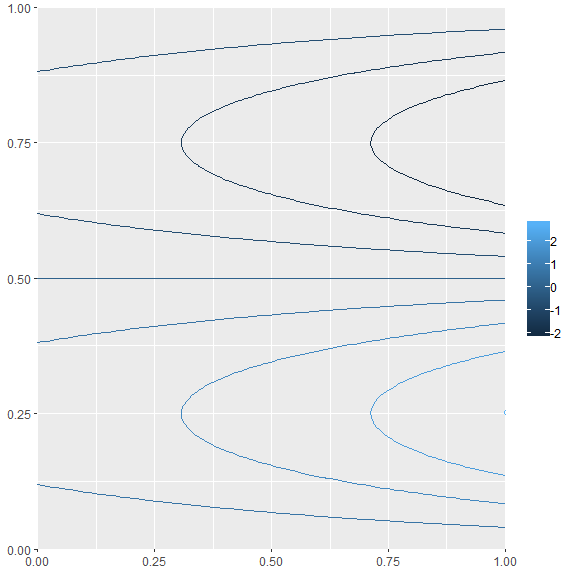Introduction to the ContourFunctions R package

2019-05-19

The ContourFunctions R package provides functions that make it easier to make contour plots. The function cf is a quick function that can take in grid data, a function, or any data, and give a contour plot showing the function or data. By default, plots are made using base graphics, but they can also be done using ggplot2.

cf_grid

cf_grid creates a contour plot from a grid of points.

Below a and b create a grid of points at which r is calculated. cf_grid is used to create the contour plot. Note that the only indication of the relationship between the colors and the r values is in the title of plot, which says that the darkest blue point is the minimum of -0.613, and the darkest pink point is the maximum of 1. (Note that this is not a good representation of the surface because there aren’t enough points in the grid, the contours are actually concentric circles as shown below.)

library(ContourFunctions)
a <- b <- seq(-4*pi, 4*pi, len = 27)
r <- sqrt(outer(a^2, b^2, "+"))
cf_grid(a, b, cos(r^2)*exp(-r/(2*pi)))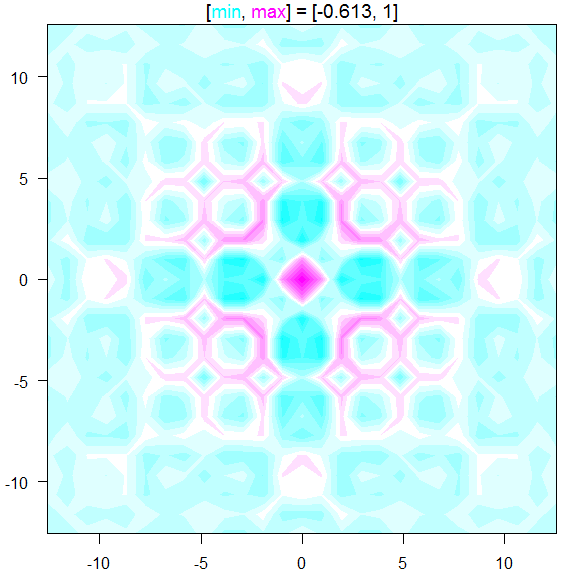To add a bar that shows how the colors relate to the output, simply set bar=TRUE, as shown below.

cf_grid(a, b, cos(r^2)*exp(-r/(2*pi)), bar=TRUE)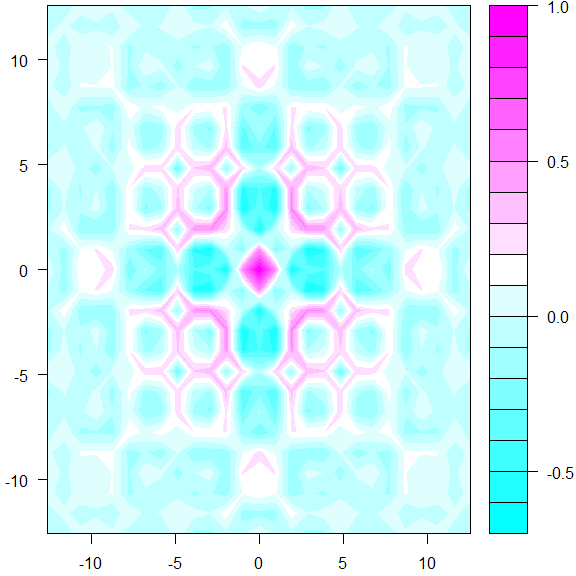Other parameters specifying details of the plot can be passed as well, see the documentation for those options.

cf_func

For the above we had to create the grid of points and give it in to cf_grid. To make this easier, cf_func allows you to simply pass in a function. It will then evaluate the function at a grid of points and pass these to cf_grid to make the contour plot.

f1 <- function(r) cos(r^2 + r^2)*exp(-sqrt(r^2 + r^2)/(2*pi))
cf_func(f1, xlim = c(-4*pi, 4*pi), ylim = c(-4*pi, 4*pi))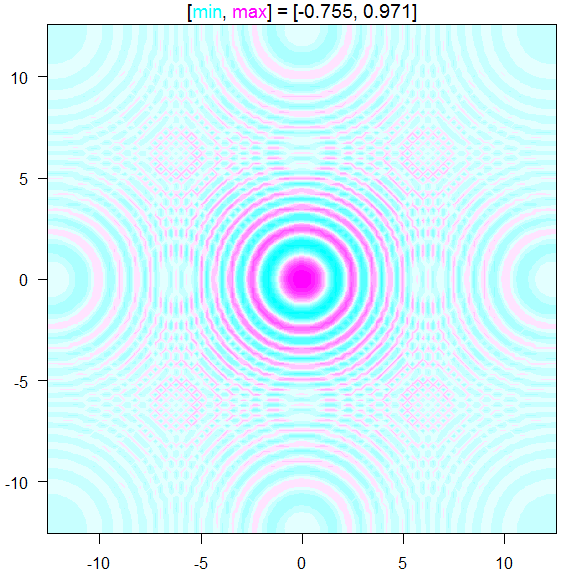If you give a function that can more efficient evaluate a bunch of points at a time, instead of one at a time, use the batchmax to have it pass points as a matrix to the given function.

The argument n controls how many points along each dimension are used. We see below that if we go back to n=27, then we get the same plot as above.

cf_func(f1, xlim = c(-4*pi, 4*pi), ylim = c(-4*pi, 4*pi), n=27)cf_data

Often one has data and wants to get an idea of what the surface looks like that fits the data. The cf_data allows the user to pass in the data to get such a plot. A Gaussian process model is fit to the data, by default using the R package laGP to do so. The model is then used to make predictions at the grid of points to make the contour plot. The model prediction function is passed to cf_func to create the contour plot. Note that this relies heavily on the model being somewhat accurate, and may not truly represent the data if the model is a poor fit.

Below a random sample of 20 points are taken from a function (a Gaussian peak centered at (0.5, 0.5)), and cf_data is used to plot the data. The black dots show the data points used to create the model.

set.seed(0)
x <- runif(20)
y <- runif(20)
z <- exp(-(x-.5)^2-5*(y-.5)^2)# + rnorm(20,0,.05)
# cf_data(x,y,z)
cf_data(x,y,z, bar=T)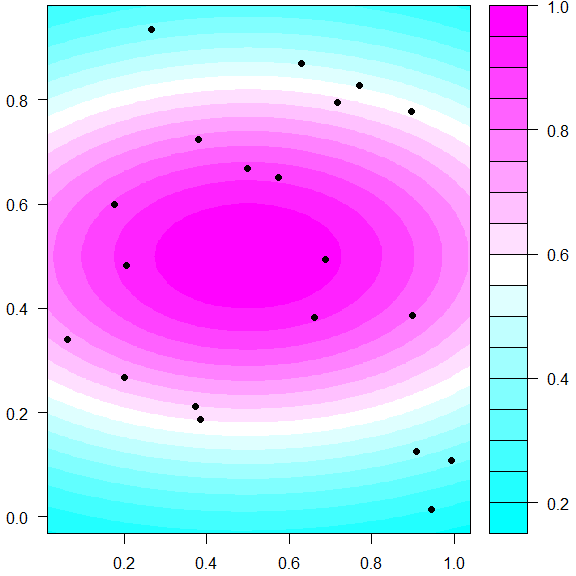afterplotfunc

The contour plots are created using the split.screen function. This causes the plot to not add additional items, such as points or lines, after making the plot. The plot below shows how when trying to add a point to the plot using points, a point that should be placed at the center ends up in the bottom right corner.

cf_func(f1, xlim = c(-4*pi, 4*pi), ylim = c(-4*pi, 4*pi))
points(c(0,0), pch=19)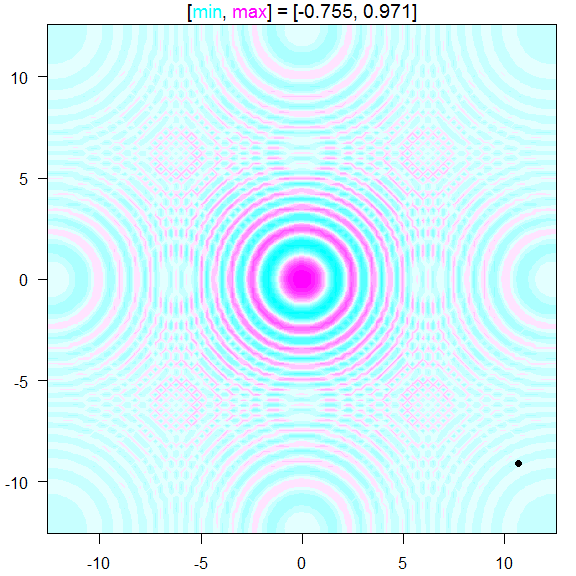If you just want to add points, you can use the parameter pts to do so. Below we see that the point ends up correctly in the center of the plot.

cf_func(f1, xlim = c(-4*pi, 4*pi), ylim = c(-4*pi, 4*pi), pts=c(0,0))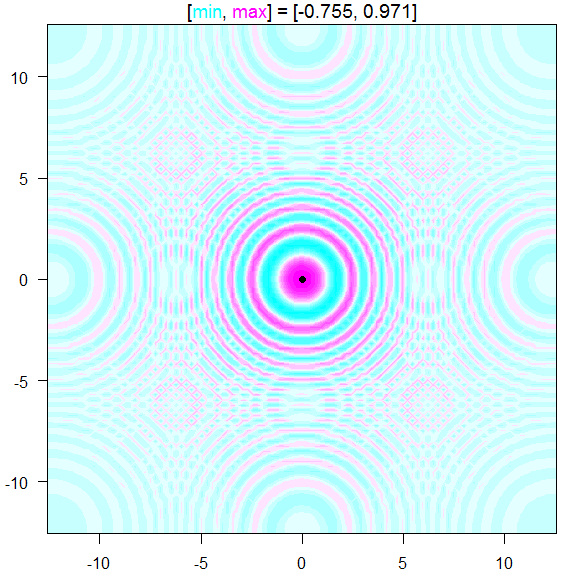Another option, that gives you more capability, is to use the parameter afterplotfunc to pass in a function that takes no arguments. After the plot is made this function will be called. You can put anything inside this function that you would normally do to a plot, including points, text, legend, and abline.

cf_func(f1, xlim = c(-4*pi, 4*pi), ylim = c(-4*pi, 4*pi),
afterplotfunc=function() {
points(5, 5, pch=19)
text(-5,5,"Text here")
legend('bottomright', legend=c(1,2,3), fill=c(1,2,3))
abline(a=0, b=1, col=2)
}
)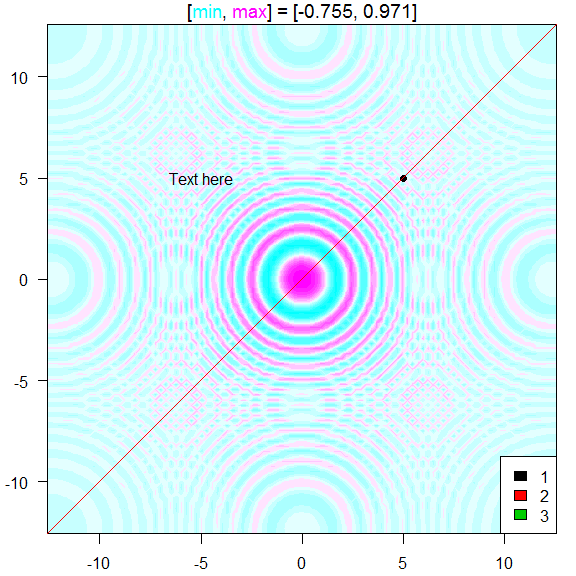cf

To make using the above cf_func and cf_data slightly easier, the same inputs can be passed to the function cf. It detects whether the first parameter is a function, in which case it passes everything to cf_func or numeric, in which case it passes everything to cf_data.

The following two plots demonstrate how cf is used. Really the only benefit is that is saves you typing _func or _grid.

cf(f1, xlim = c(-4*pi, 4*pi), ylim = c(-4*pi, 4*pi))cf(x,y,z, bar=T)cf_highdim

For higher dimensional functions, cf_highdim makes a contour plot of two-dimensional slices of the given function. The dimensions not being shown can be set to a default value or averaged out.

friedman <- function(x) {
10*sin(pi*x*x) + 20*(x-.5)^2 + 10*x + 5*x
}
cf_highdim(friedman, 5, color.palette=topo.colors)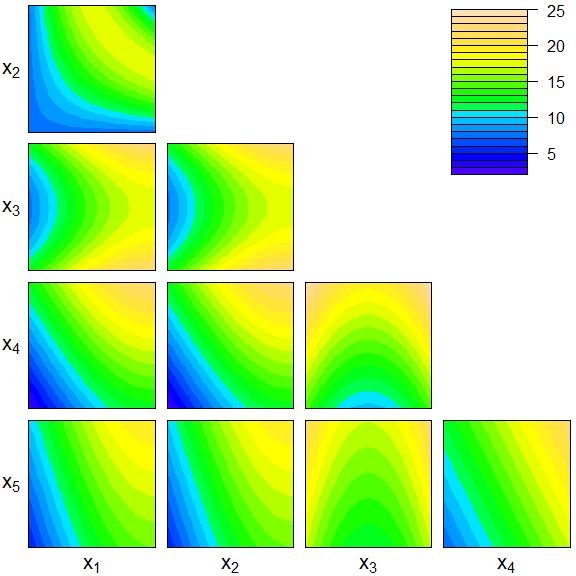cf_4dim

Functions with four input dimensions can be displayed using a grid of contour plots with the function cf_4dim. Two of the dimensions are shown on each plot, while the other two are set to a specific value for the given plot.

cf_4dim(function(x) {x + x^2 + sin(2*pi*x)})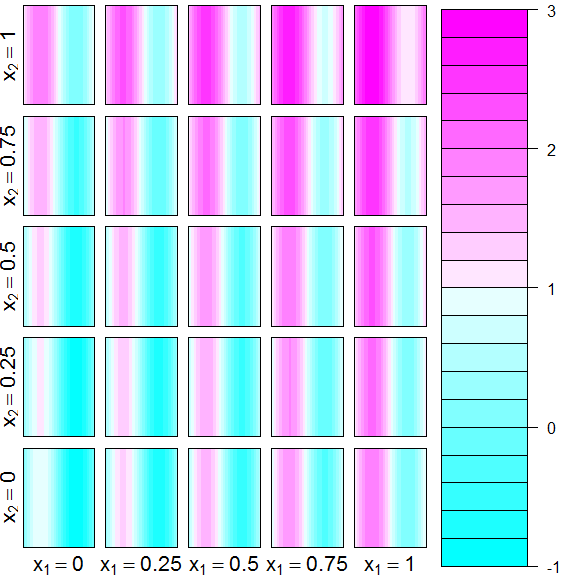Making plots with ggplot2

All of the above plots used R base graphics. Similar functions for cf, cf_func, cf_data, and cf_grid that use ggplot2 are also available as gcf, gcf_func, gcf_data, and gcf_grid

f2 <- function(x) {exp(x) * sin(2*pi*x)}
gcf(f2)
## Registered S3 methods overwritten by 'ggplot2':
##   method         from
##   [.quosures     rlang
##   c.quosures     rlang
##   print.quosures rlang